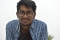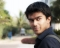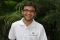## Armstrong number in Java.Published on 23-Apr-2018 07:46:53
Following is the required program. Live Demopublic class Tester { public static void main(String[] args) { int c = 0, a, temp; int m = 153; int n = m; ... Read More

## Factorial program in Java without using recursion.Published on 23-Apr-2018 07:42:19
Following is the required program.ExampleLive Demopublic class Tester {    static int factorial(int n) {       if (n == 0)          return 1;       else          return (n * factorial(n - 1));    }    public static void main(String args[]) ... Read More

## Factorial program in Java using recursion.Published on 22-Apr-2018 19:15:58
Following is the required program.ExampleLive Demopublic class Tester {    public static void main(String args[]) {       int i, fact = 1;       int number = 5;       for (i = 1; i <= number; i++) {          fact = fact ... Read More

## Palindrome program in Java.Published on 22-Apr-2018 19:08:15
Following is the required program.ExampleLive Demopublic class Tester {    public static void main(String args[]) {       int r, sum = 0, temp;       int m = 454;       int n = m;       temp = n;       while (n ... Read More

## Prime number program in Java.Published on 22-Apr-2018 19:02:28
Following is the required program.ExampleLive Demopublic class Tester {    public static void main(String args[]) {       int i, m = 0, flag = 0;       int n = 41;// it is the number to be checked       m = n / 2;   ... Read More

## Fibonacci series program in Java using recursion.Published on 22-Apr-2018 19:11:48
Following is the required program.ExampleLive Demopublic class Tester {    static int n1 = 0, n2 = 1, n3 = 0;    static void fibbonacci(int count) {       if (count > 0) {          n3 = n1 + n2;          n1 = ... Read More

## Fibonacci series program in Java without using recursion.Published on 22-Apr-2018 18:07:48
Following is the required program.ExampleLive Demopublic class Tester {    public static void main(String args[]) {        int n1 = 0, n2 = 1, n3, i, max = 5;        System.out.print(n1 + " " + n2);        for (i = 2; i < max; ... Read MorePublished on 22-Apr-2018 18:13:03
/** is known as documentation comments. It is used by javadoc tool while creating the documentation for the program code./**    * This is a documentation comment.    * This is my first Java program.    * This will print 'Hello World' as the output    * This is an ... Read More

## How to randomize (shuffle) a JavaScript array?Published on 22-Apr-2018 18:56:46
To randomize a JavaScript array to display random elements, you can try to run the following code:ExampleLive Demo<html>        <body>                     <script>            function randomFunc(myArr) {                   ... Read More

## How to extend an existing JavaScript array with another array?Published on 22-Apr-2018 18:52:16
To extend an existing array in JavaScript, use the Array.concat() method.ExampleYou can try to run the following code to extend an existing JavaScript array with another array:Live Demo<html>        <head>             <title>JavaScript Array push Method</title>        </head>         ... Read More